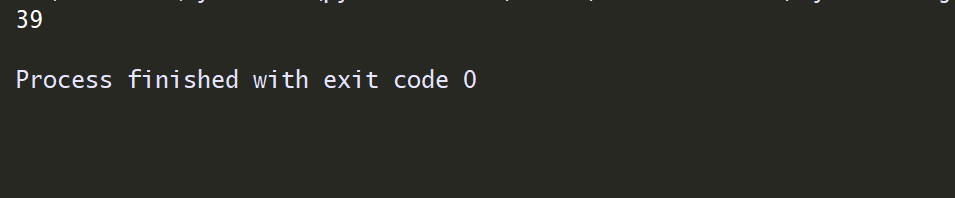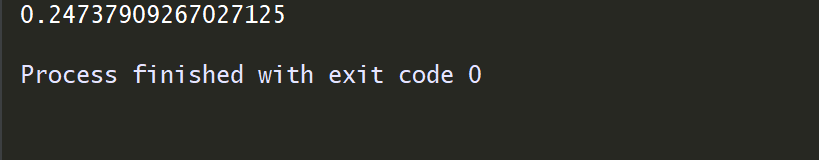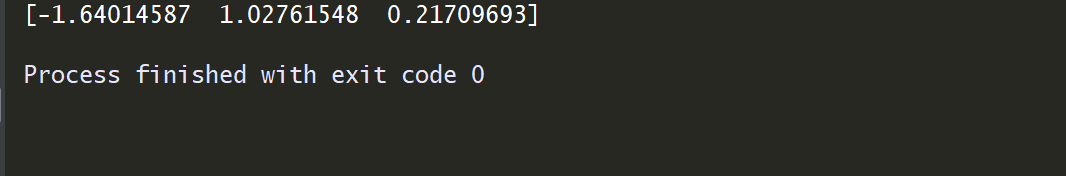• 在JDK的java.util包中有一个Random类，他可以在指定的取值范围内随机产生数字。在Random类中有两种构造方法 使用方式: import导包：所属包java.util.Random 创建实例格式：Random 变量名 = new Random()； 1、...
在JDK的java.util包中有一个Random类，他可以在指定的取值范围内随机产生数字。在Random类中有两种构造方法

使用方式:

import导包：所属包java.util.Random

创建实例格式：Random 变量名 = new Random()；

1、random.nextInt()

不带参数的nextInt()会生成所有有效的整数（包含正数，负数，0）

带参的nextInt(int x)则会生成一个范围在0~x（不包含X）内的任意正整数，

例如：int x=new Random.nextInt(100);  则x为一个0~99的任意整数

int x=new Random.nextInt(100)+1  则x为一个1~100的任意整数

带参的nextInt(max)%(max-min+1)+min 则会生成一个指定范围内的整数

        Random ran = new Random();// 随机数类
Set<Integer> set = new HashSet<Integer>();
//  set.size()==10用来确定获取的数据共有多少个
while(set.size()==10?false:true){
int num = ran.nextInt(100);
}
Iterator<Integer> it = set.iterator();
int count = 0;
while(it.hasNext()){
count++;
System.out.println("第"+count +"个随机数:"+it.next());
}

2、random.nextBytes()

用于生成随机 byte 值，再将其放入指定数组长度的byte 数组中

        Random random = new Random();
byte[] data = new byte;
random.nextBytes(data);
System.out.println(Arrays.toString(data)); // [-73, 18, -110, -79, 56]

3、random.nextDouble()  [ nextFloat() 同理】

用于从该随机数生成器的序列中得到下一个伪均匀分布在 0.0 到 1.0 之间的 double 值，[0.0, 1.0)

Random random = new Random();
System.out.println(random.nextDouble()); // 0.15068131311276745



展开全文• python中生成随机整数，随机小数，0-1之间的小数 1.生成随机整数 import random print(random.randint(1,100))#生成1到100之间的随机整数 2.生成0-1之间随机的小数 import numpy as np print(random....
python中生成随机整数，随机小数，0-1之间的小数

1.生成随机整数

import random

print(random.randint(1,100))#生成1到100之间的随机整数2.生成0-1之间随机的小数

import numpy as np

print(random.random())3.生成随机小数，randn(3)代表生成三个

import numpy as np

print(np.random.randn(3))展开全文• VB 产生随机整数过程 VB 产生随机整数过程 VB 产生随机整数过程
• ## 生成随机整数方法

千次阅读 2014-09-18 14:49:00
rand()函数常用来生成0-1之间的随机矩阵，那么我们完全也可以用来生成我们想要的任意大小、任意个随机整数。 1，生成0-5之间的10个随机整数 在matlab主窗口中输入round(rand(1,10)*5)

MATLAB 生成随机整数 的各种方法以及实例

MATLAB提供了多种生成随机整数的方法，下面我们就着重介绍几种常用的方法。

方法一：rand()
rand()函数常用来生成0-1之间的随机矩阵，那么我们完全也可以用来生成我们想要的任意大小、任意个数的随机整数。
1，生成0-5之间的10个随机整数   在matlab主窗口中输入round(rand(1,10)*5) 回车2，生成1个随机整数，命令  round(rand(1,1)*5)  回车3，生成-5-4之间的10个随机整数  在matlab主窗口中输入   round(rand(1,10)*9)-5  回车我们可以看到生成的是在-5到4之间的随机整数！

方法二： randint()
randint()函数可以生成任意区间任意维数的随机整数矩阵，下面我们就举例验证之.
1,生成1个5-9之间的随机整数在主窗口中输入 randint(1,1,[5 9])回车2，生成10个5到20之间的整数在主窗口中输入  randint(1,10,[10 20])  回车黄色警告的意思是这个函数未来可能会被MATLAB移除，请用rand()函数，当然我们现在不熟悉rand()函数，还可以继续用这个函数。
3，生成10个-7到15之间的随机整数在主窗口中输入  randint(1,10,[-7 15]) 回车结论：randint()函数其实是rand()函数的特殊版本，randint()能够生成的所有整数rand()函数都可以做到，不过需要我们自己去手动进行取整以及区间变换操作，randint()函数用起来方便，但是未来有可能这个函数被删除，所有希望大家也多去了解一下rand()函数。

展开全文• python中生成随机整数Following are the few explanatory illustrations using different python modules, on how to generate random integers? Consider the scenario of generating the random numbers between 0...
python中生成随机整数Following are the few explanatory illustrations using different python modules, on how to generate random integers? Consider the scenario of generating the random numbers between 0 and 9 (both inclusive).
以下是使用不同的python模块的一些说明性插图，说明如何生成随机整数？ 考虑生成介于0和9之间(包括两端)的随机数的方案。
使用randrange (Using randrange )
Syntax:
句法：
    random.randrange(stop)
random.randrange(start, stop, step)

Code:
码：
>>> import random
>>> for i in range(10):
...     print(random.randrange(10))
...
2
2
2
0
8
8
5
6
6
3

使用randint (Using randint)
Syntax:
句法：
    random.randint(a,b)

Code:
码：
>>> import random
>>> for i in range(10):
...     print(random.randint(0,10))
...
1
6
7
5
8
9
6
2
3
9
>>>

使用机密 (Using secrets)
By using this method, we can generate cryptographically strong random numbers.
通过使用此方法，我们可以生成加密强度高的随机数。
>>> from secrets import randbelow
>>> for i in range(10):
...     print(randbelow(10))
...
6
5
2
0
7
2
0
1
2
6
>>>


翻译自: https://www.includehelp.com/python/generate-random-integers-between-0-and-9-in-python.aspxpython中生成随机整数
展开全文python random numpy java 机器学习
• ## python生成随机整数

千次阅读 2019-10-08 19:09:48
python生成随机不重复的整数，用random中的sample index = random.sample(range(0,10),10) 上面是生成不重复的10个从1~10的整数 python生成完全随机整数，用numpy中的random.randint index = np....
• ## numpy生成随机整数

千次阅读 2019-11-28 11:04:41
np.random.randint(0,999) 随机生成0-999的整数
•java random import string class
• php随机生成小数或整数 随机整数生成 (Random integer number generation ) To generate a random integer, we can use random_int() function, which is a library function in PHP, it accepts two arguments ...
• mt_rand() 函数使用 Mersenne Twister 算法生成随机整数。 提示：该函数是产生随机值的更好选择，返回结果的速度是 rand() 函数的 4 倍。 提示：如果您想要一个介于 10 和 100 之间（包括 10 和 100）的随机...
• ## MySQL生成随机整数

千次阅读 2015-09-04 11:49:32
若要在i ≤ R ≤ j 这个范围得到一个随机整数R ，需要用到表达式FLOOR(i + RAND() *(j – i +1))。 例如， 若要在7 到 12的范围（包括7和12）内得到一个随机整数, 可使用以下语句： SELECTFLOOR(7 +...MySQL
• ## JS生成随机整数

千次阅读 2018-06-20 15:52:13
用JS的随机数生成函数random()配合其他数学函数可以限制随机数的取值。 JS的随机数函数及相关函数： Math.random(); 结果为0-1间的一个随机数(包括0,不包括1) 。 Math.floor(num); 参数num为一个数值，函数结果为...
• ## Android生成随机整数

千次阅读 2018-10-24 19:16:56
package cc.test; import java.util.HashSet; import java.util.Random; import android.app.Activity; import android.os.Bundle;... * Java中利用Random生成随机数 * */ public class TestRandomAc...随机数
• rand()函数常用来生成0-1之间的随机矩阵，那么我们完全也可以用来生成我们想要的任意大小、任意个随机整数。 1，生成0-5之间的10个随机整数 在matlab主窗口中输入round(rand(1,10)*5) 回车2，生成1个随机...
• 生成单个随机整数 import random '''方法1''' rand_int=random.randint(0,100) '''方法2''' rand_int_c=random.choice(range(0,10)) print(rand_int) print(rand_int_c) 生成单个随机小数 import random rand_...
• 首先使用np.random生成随机数，然后变成dataframe 即可 import pandas as pd import numpy as np df1 = pd.DataFrame(np.random.randint(0,2,size=(100, 1)),columns=['test']) # 生成0，1序列，并指定名称为'test'...python
• Oracle生成某区间内随机整数的方法可以用以下两种方式： Select round(dbms_random.value(0, 100), 0) as 随机数一, trunc(dbms_Random.Value(0, 100)) as 随机数二 From dual 其中 round和trunc的区别是：前者...
• C#编写的生成随机数的程序，给定范围，生成范围之内的随机整数，也可以生成两位小数
• /** * java生成随机数字10位数 * * @param length[生成随机数的长度] * @return */ public static String getRandomNickname(int length) { String val = ""; Random random = new...java random
• 需求： 使用JavaScript生成随机位数的字母数字组合和指定位数字母数字组合。 代码： 此代码可以放到你的公共方法里，需要用的时候引入使用... * @param {number} min 生成随机位数的最小 * @param {number} ...
• 生成随机整数:可以调用random中的randint(a,b)括号中的是你想要填的随意区间随机小数：random.randn()这个括号填的是你要随机取小数的个数完整代码 import random import numpy as np a ...
• 第一种： //创建随机数类的对象 Random rd=new Random(); for (int i = 0; i < 7; i++) { ... System.out.println("生成33以内的随机整数:"+(rd.nextInt(33)+1)); } 第二种： ...
• 在实际开发需求中，需要生成0到某个之间的随机整数： Math.floor(Math.random()*10) 结合Math.floor()向下取整，能给我们随机生成0-9之间的整数。 但是请注意，上面只能生成0到某个之间的随机数，但我们可以...
• update 表名 set 列名 = (select cast(ceiling(rand(checksum(newid()))*随机数的范围)as int ))例如： update tb_News set Clickvolume = (select cast(ceiling(rand(checksum(newid...在tb_News中的Clickvolume生成1sql
• 1. 使用Random类的nextInt方法： Random rand = new Random(); rand.nextInt(max);, 此时输出[0,max),注意右边是开区间，如果需要设定最小值可通过 rand.nextInt(max-min+1)+min方式，此时的范围为[min,max] ......